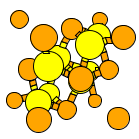Crystal Lattice Structures: Creation Date: 5 May 2002 Last Modified: 21 Oct 2004

# The FeS2 (P1) StructureYou can now

• This structure is just a slightly distorted version of Pyrite (C2), with no rotational symmetry whatsoever,
• Even though the unit cell is cubic: a=b=c, α=β=γ=90°.

• Prototype: FeS2
• Pearson Symbol: aP12
• Space Group: P1 (Cartesian and lattice coordinate listings available)
• Number: 1
• Primitive Vectors:  A1 = a X A2 = bx X + by Y A3 = cx X + cy Y + cz Z

In terms of the traditional lattice parameters: a, b, c, α, β, and γ:

 bx = b cos(γ) by = b sin(γ) cx = c cos(β) cy = c [ cos(α) - cos(β) cos(γ) ] / sin(γ) cz = [c2 - cx2 - cy2]½
• Basis Vectors:
• All the atoms, of course, are on (1a) sites. Atoms 1-4 are Fe, Atoms 5-12 S:

 Bi = + x A1 + y A2 + z A3 = + (x a + y bx + z cx) X + (y by + z cy) Y + z cz Z (1a) [i = 1, 2, 3, ... , 12]

Go back to the Sulfur and its compounds page.

Go back to Crystal Lattice Structure page.

 Structures indexed by: This is a mirror of an old page created at theNaval Research LaboratoryCenter for Computational Materials ScienceThe maintained successor is hosted at http://www.aflowlib.org/CrystalDatabase/ and published as M. Mehl et al., Comput. Mater. Sci. 136 (Supp.), S1-S828 (2017).